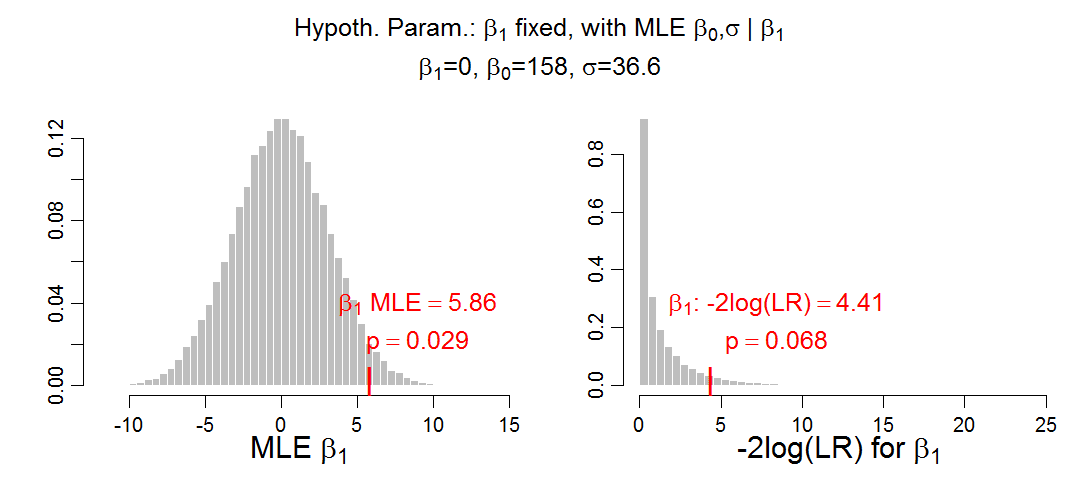## Wednesday, September 17, 2014

### Sampling distribution of maximum lilkelihood estimate - help?

I'm doing Monte Carlo simulation of sampling distributions to compute p values. For example, consider the slope parameter, β1, in simple linear regression. I want to find out if p < .05 for a null hypothesis that β1=0. I'm working with two different ways to compute a p value, and am finding that the results do not agree with each other. Here are the two ways:
1. Consider the maximum likelihood estimate (MLE) of β1 from the actual data, denoted β1MLEactual, and see where it falls in a sampling distribution of β1MLEnull for simulated data from the null hypothesis.
2. Consider the likelihood ratio statistic, G2 = -2log(LR) where LR is the ratio of the maximum likelihood of the restricted model with β1=0 over the maximum likelihood of the full model with β1 free. We see where G2actual falls in the sampling distribution of G2null for simulated data from the null hypothesis.
I'm finding that the p values don't agree. What am I doing wrong?

Here's an example with details. Consider the data in this scatter plot:
The maximum-likelihood regression line is shown above in blue, and the maximum-likelihood zero-slope line is shown in red. The MLE parameter values are shown in the header of the plot; in particular β1MLEactual = 5.86. The header also shows that G2actual = 4.41.

For reference, in R a call to lm yields p = 0.068 for the slope coefficient. Let's see if either of the Monte Carlo schemes will agree with that result.

We need to generate Monte Carlo data samples. For this purpose, I use the x values in the actual data, and for each x value I generate a random y value according to the MLE values of the restricted model. The restricted model has parameter values shown in the header of the figure below (rounded to three digits):Sampling distributions from the null hypothesis. The null-hypothesis parameter values are shown in the header of the
Thus, for each x value in the data, a random value for y is created in R as rnorm(1,mean=158,sd=36.6)using the full-precision MLE values, not just the rounded values shown here. For each simulated set of data, I (well, the computer) computed β1MLEnull and G2null. There were 50,000 simulated data sets to create fairly smooth and stable sampling distributions, shown above.

The sampling distributions above also show the locations of β1MLEactual and G2actual along with their p values. You can see that the p value for β1MLEactual does not match the p value for G2actual, but the latter does match the result of R's lm function.

Maybe the problem is merely Monte Carlo sampling noise. But, no, even much bigger simulations don't help (and the p values stay essentially the same).

Maybe the problem is one-tailed vs two-tailed p values. But, no, two times the MLE p value does not equal the G2 p value.

Maybe the problem is that the null hypothesis σ should be the unbiased estimate of SD(y), using N-1 in the denominator for computing SD, instead of the MLE for SD(y), which effectively uses N in the denominator. But, no, that still doesn't make the p value match. The p value for β1MLE does increase (i.e., the sampling distribution of β1MLEnull widens while the sampling distribution of G2null remains unchanged), but still does not match, either one-tailed or two-tailed.

What am I doing wrong with the sampling distribution of  β1MLEnull?